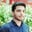Related Tags

bitwise_and
numpy
python
communitycreator

# What is bitwise_and() in NumPy?Salman Yousaf

### Overview

The NumPy bitwise_and() method is used to evaluate bitwise logical AND operators between two arrays. It performs the bitwise logical AND operation of underlying binary representations.

### Syntax

 numpy.bitwise_and(arr1,
arr2,
out=None,
where=True,
**kwargs)

### Parameters

It takes the following argument values:

• arr1: This is an array-like object. It only handles integer and boolean-type arrays.
• arr2: This is an array-like object. It only handles integer and boolean-type arrays.
• out: This is the memory location in which the result will be stored. It will be of the same dimension as arr1 or arr2. If it is set to None, a new array of the same dimensions will be initialized.
• where: This is an optional parameter. If its value is True, then the result of the function will be replaced with the ufunc result. If set to False, the original value will be returned as it is. Its default value is True.
• **kwargs: These are additional arguments.

### Return value

It returns ndarray or scalar type objects.

### Code

In this code, we'll take a look at numpy.bitwise_and() with different argument values.

# importing numpy library
import numpy as np
# performing logical AND between 12, 16
print("12 AND 16:", end=" ")
print(np.bitwise_and(12, 16))
# logical AND between a Python list [3,13] and 12
print("[3,13] AND 12:", end=" ")
print(np.bitwise_and([3,13], 12))
# logical AND between two lists of same size
print("[9,7] AND [8,35]:", end=" ")
print(np.bitwise_and([9,7], [8,35]))
# performing logical AND between two numpy arrays
print("np.array([2,7,255]) AND  np.array([6,12,18]):", end=" ")
print(np.bitwise_and(np.array([2,7,255]), np.array([6,12,18])))

### Code explanation

• Lines 4–5: We perform bitwise AND between 12 (0000 1100) and 16 (0001 0000). This will return 0.
• Lines 7–8: We perform bitwise AND between a list [3,13]=>(0000 0011, 0000 1101) and 12 (0000 1100). This will return a list.
• Lines 10–11: We perform bitwise AND between 2 lists [9,7] and [8,35].
• Lines 13–14: We perform bitwise AND between two Numpy arrays np.array([2,7,255]) and np.array([6,12,18].

### Implementation of ufunc

Numpy also supports universal functions (ufunc) that implement C or core Python operator &.

# importing numpy library
import numpy as np
# creating two numpy arrays
x1 = np.array([4, 7, 255])
x2 = np.array([8,13,19])
# performing logical AND using conventional & operator
print(x1 & x2)

### Explanation

• Line 4: We create a NumPy array x1 containing [4, 7, 255].
• Line 5: We create a NumPy array x2 containing [8,13,19].
• Line 7: We perform logical AND by using a conventional & operator.

RELATED TAGS

bitwise_and
numpy
python
communitycreator

CONTRIBUTORSalman Yousaf
RELATED COURSES

View all Courses

Keep Exploring

Learn in-demand tech skills in half the time